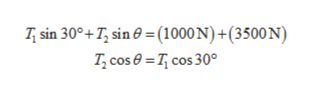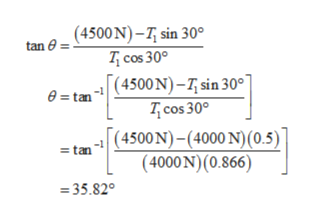# A 3-meter rod with a weight of 1000 N is suspended by two cables and has a large box(3500N in weight ) tied to the right-hand side hanging vertically. The center of mass of the rod is .5min from the leftmost edge. One cable is exactly on the leftmost end of the rod with a tension of4000 N at an angle of 30 degrees to the rod . The second cable is 1 m to the right of the center ofmass and has an unknown tension. What is the tension in the second cable and what angledoes it make with the rod? You must draw a diagram so I can understand your answer.

Question
24 views

A 3-meter rod with a weight of 1000 N is suspended by two cables and has a large box(3500
N in weight ) tied to the right-hand side hanging vertically. The center of mass of the rod is .5
min from the leftmost edge. One cable is exactly on the leftmost end of the rod with a tension of
4000 N at an angle of 30 degrees to the rod . The second cable is 1 m to the right of the center of
mass and has an unknown tension. What is the tension in the second cable and what angle
does it make with the rod? You must draw a diagram so I can understand your answer.

check_circle

Step 1

Consider the free body diagram below.

Step 2

Using the free body diagram,help_outlineImage Transcriptionclose(1000 N) + (3500 N) T, sin 30°+7, sin T, cose = T cos 30° fullscreen
Step 3

Therefore, the angle made by tension i...help_outlineImage Transcriptionclose(4500 N)-7 sin 30° cos 30° (4500 N)-7, sin 30° tan e=tan T, cos 30 (4500 N)-(4000 N)(0.5) (4000 N) (0.866) =tan =35.820 fullscreen

### Want to see the full answer?

See Solution

#### Want to see this answer and more?

Solutions are written by subject experts who are available 24/7. Questions are typically answered within 1 hour.*

See Solution
*Response times may vary by subject and question.
Tagged in

### Kinematics